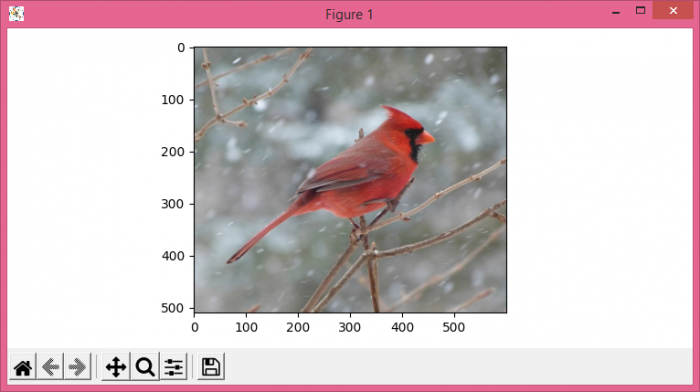# How to remove grid lines from an image in Python Matplotlib?

To remove grid lines from an image, we can take the following steps −

• Set the figure size and adjust the padding between and around the subplots.
• Load an image from a file.
• Convert the image from one color space to another.
• To remove grid lines, use ax.grid(False).
• Display the data as an image, i.e., on a 2D regular raster.
• To display the figure, use show() method.

## Example

from matplotlib import pyplot as plt
import cv2

plt.rcParams["figure.figsize"] = [7.50, 3.50]
plt.rcParams["figure.autolayout"] = True

plt.show()